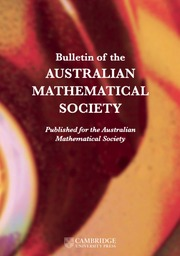Home
Hostname: page-component-768dbb666b-qc52z Total loading time: 0.596 Render date: 2023-02-05T15:23:46.003Z Has data issue: true Feature Flags: { "useRatesEcommerce": false } hasContentIssue trueBulletin of the Australian Mathematical Society

## Abstract

For a fixed integer h, the standard orthogonality relations for Ramanujan sums$c_r(n)$ give an asymptotic formula for the shifted convolution$\sum _{n\le N} c_q(n)c_r(n+h)$ . We prove a generalised formula for affine convolutions$\sum _{n\le N} c_q(n)c_r(kn+h)$ . This allows us to study affine convolutions$\sum _{n\le N} f(n)g(kn+h)$ of arithmetical functions$f,g$ admitting a suitable Ramanujan–Fourier expansion. As an application, we give a heuristic justification of the Hardy–Littlewood conjectural asymptotic formula for counting Sophie Germain primes.

## MSC classification

Type
Research Article
© The Author(s), 2022. Published by Cambridge University Press on behalf of Australian Mathematical Publishing Association Inc.

## Access options

Get access to the full version of this content by using one of the access options below. (Log in options will check for institutional or personal access. Content may require purchase if you do not have access.)

## References

Carmichael, R. D., ‘Expansions of arithmetical functions in infinite series’, Proc. Lond. Math. Soc. (2) 34 (1932), 126.CrossRefGoogle Scholar
Coppola, G. and Murty, M. R., ‘Finite Ramanujan expansions and shifted convolution sums of arithmetical functions, II’, J. Number Theory 185 (2018), 1647.CrossRefGoogle Scholar
Coppola, G., Murty, M. R. and Saha, B., ‘On the error term in a Parseval type formula in the theory of Ramanujan expansions II’, J. Number Theory 160 (2016), 700715.CrossRefGoogle Scholar
Coppola, G., Murty, M. R. and Saha, B., ‘Finite Ramanujan expansions and shifted convolution sums of arithmetical functions’, J. Number Theory 174 (2017), 7892.CrossRefGoogle Scholar
Gadiyar, H. G., Murty, M. R. and Padma, R., ‘Ramanujan–Fourier series and a theorem of Ingham’, Indian J. Pure Appl. Math. 45(5) (2014), 691706.CrossRefGoogle Scholar
Gadiyar, H. G. and Padma, R., ‘Ramanujan–Fourier series, the Wiener–Khintchine formula and the distribution of prime pairs’, Phys. A 269(2–4) (1999), 503510.CrossRefGoogle Scholar
Hardy, G. H., ‘Note on Ramanujan’s trigonometrical function${c}_q(n)$ and certain series of arithmetical functions’, Math. Proc. Cambridge Philos. Soc. 20 (1921), 263271.Google Scholar
Ingham, A. E., ‘Some asymptotic formulae in the theory of numbers’, J. Lond. Math. Soc. (2) 2 (1927), 202208.CrossRefGoogle Scholar
Murty, M. R., ‘Ramanujan series for arithmetical functions’, Hardy–Ramanujan J. 36 (2013), 2133.Google Scholar
Murty, M. R. and Saha, B., ‘On the error term in a Parseval type formula in the theory of Ramanujan expansions’, J. Number Theory 156 (2015), 125134.CrossRefGoogle Scholar
Ramanujan, S., ‘On certain trigonometric sums and their applications in the theory of numbers’, Trans. Cambridge Philos. Soc. 22 (1918), 179199.Google Scholar
Saha, B., ‘A note on arithmetical functions with absolutely convergent Ramanujan expansions’, Int. J. Number Theory 12(6) (2016), 15951611.CrossRefGoogle Scholar

# Save article to Kindle

Note you can select to save to either the @free.kindle.com or @kindle.com variations. ‘@free.kindle.com’ emails are free but can only be saved to your device when it is connected to wi-fi. ‘@kindle.com’ emails can be delivered even when you are not connected to wi-fi, but note that service fees apply.

Find out more about the Kindle Personal Document Service.

AFFINE CONVOLUTIONS, RAMANUJAN–FOURIER EXPANSIONS AND SOPHIE GERMAIN PRIMES
Available formats
×

# Save article to Dropbox

To save this article to your Dropbox account, please select one or more formats and confirm that you agree to abide by our usage policies. If this is the first time you used this feature, you will be asked to authorise Cambridge Core to connect with your Dropbox account. Find out more about saving content to Dropbox.

AFFINE CONVOLUTIONS, RAMANUJAN–FOURIER EXPANSIONS AND SOPHIE GERMAIN PRIMES
Available formats
×

# Save article to Google Drive

To save this article to your Google Drive account, please select one or more formats and confirm that you agree to abide by our usage policies. If this is the first time you used this feature, you will be asked to authorise Cambridge Core to connect with your Google Drive account. Find out more about saving content to Google Drive.

AFFINE CONVOLUTIONS, RAMANUJAN–FOURIER EXPANSIONS AND SOPHIE GERMAIN PRIMES
Available formats
×
×